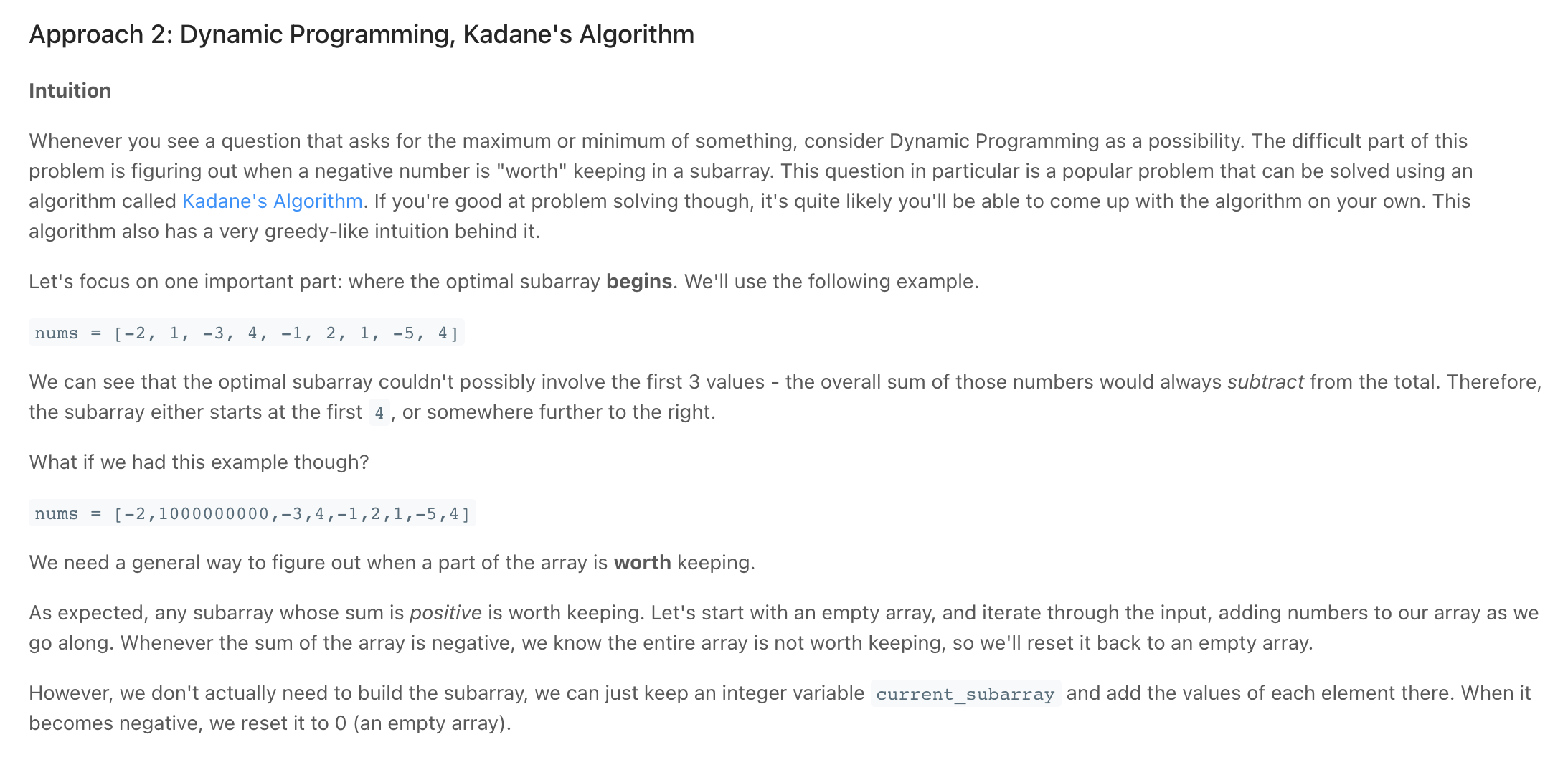# Maximum SubarrayScreen Recording 2021-11-01 at 15.30.10.mov

"""
Maximum Subarray:

Given an integer array nums, find the contiguous subarray (containing at least one number) which has the largest sum and return its sum.
Follow up: If you have figured out the O(n) solution, try coding another solution using the divide and conquer approach, which is more subtle

Example 1:
Input: nums = [-2,1,-3,4,-1,2,1,-5,4]
Output: 6
Explanation: [4,-1,2,1] has the largest sum = 6.
Example 2:
Input: nums = 
Output: 1
Example 3:
Input: nums = [5,4,-1,7,8]
Output: 23

https://leetcode.com/problems/maximum-subarray/
"""

from typing import List

# O(n) time | O(1) space
class Solution:
def maxSubArray(self, nums: List[int]):
# # # find the maximum subarray per given element:
# # check which one is larger:
# # adding the element to the current subarray or starting a new subarray at the element

# the max subarray we found's sum
max_sa_sum = float("-inf")

# sum of the current subarray that we are working with
curr_subarray = float("-inf")
for num in nums:

# check if adding the num to the current subarray will be
# a longer sum than starting a new subarray at the element
# then the current subarray should be the longer/larger of the two
curr_subarray = max(num, curr_subarray + num)

# record the largest (sum) we found
max_sa_sum = max(curr_subarray, max_sa_sum)

return max_sa_sum

"""
Inputs:
[-2,1,-3,4,-1,2,1,-5,4]
[-2]

[-2,-3,-1,-5]
[1,2,3,4,5,6,7,8,9,0]
Outputs:
6
-2
1
-1
45
"""